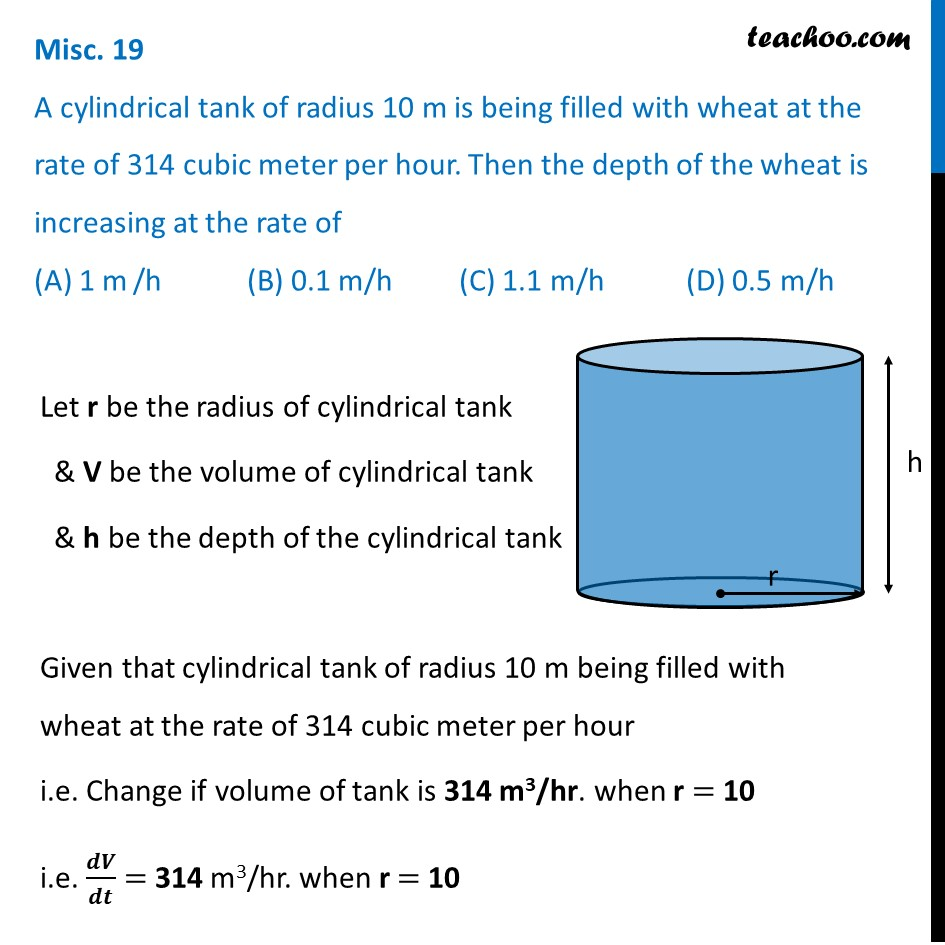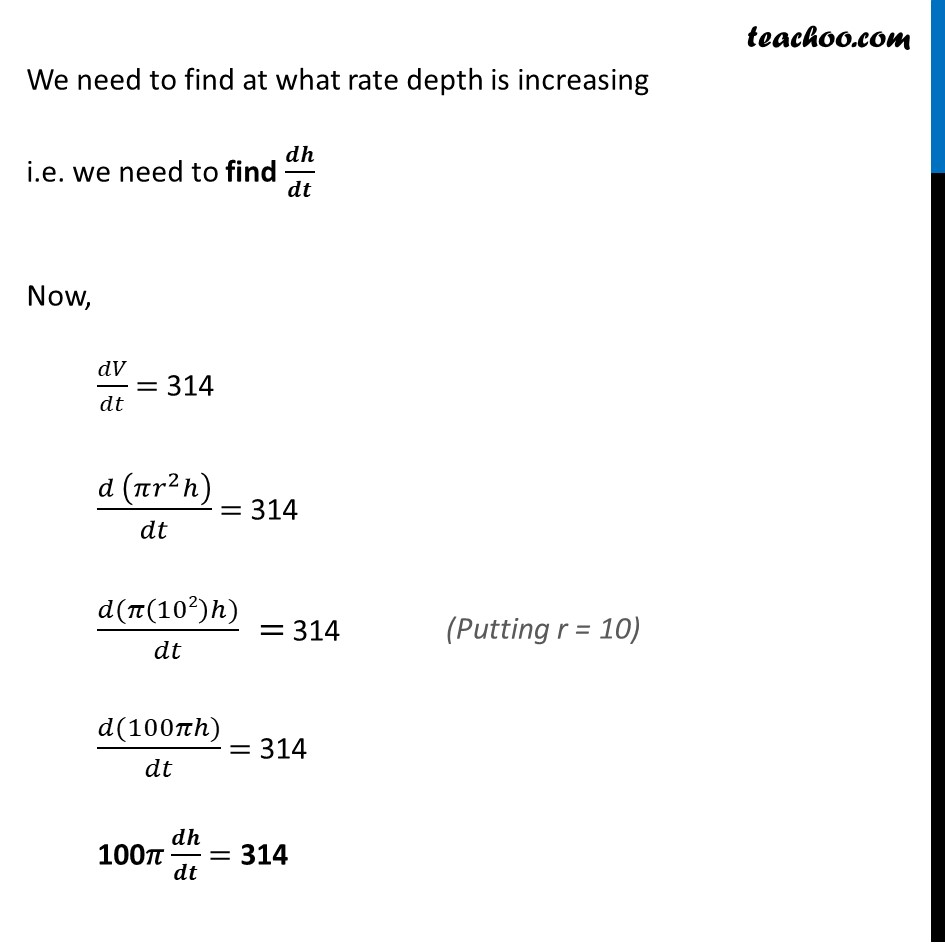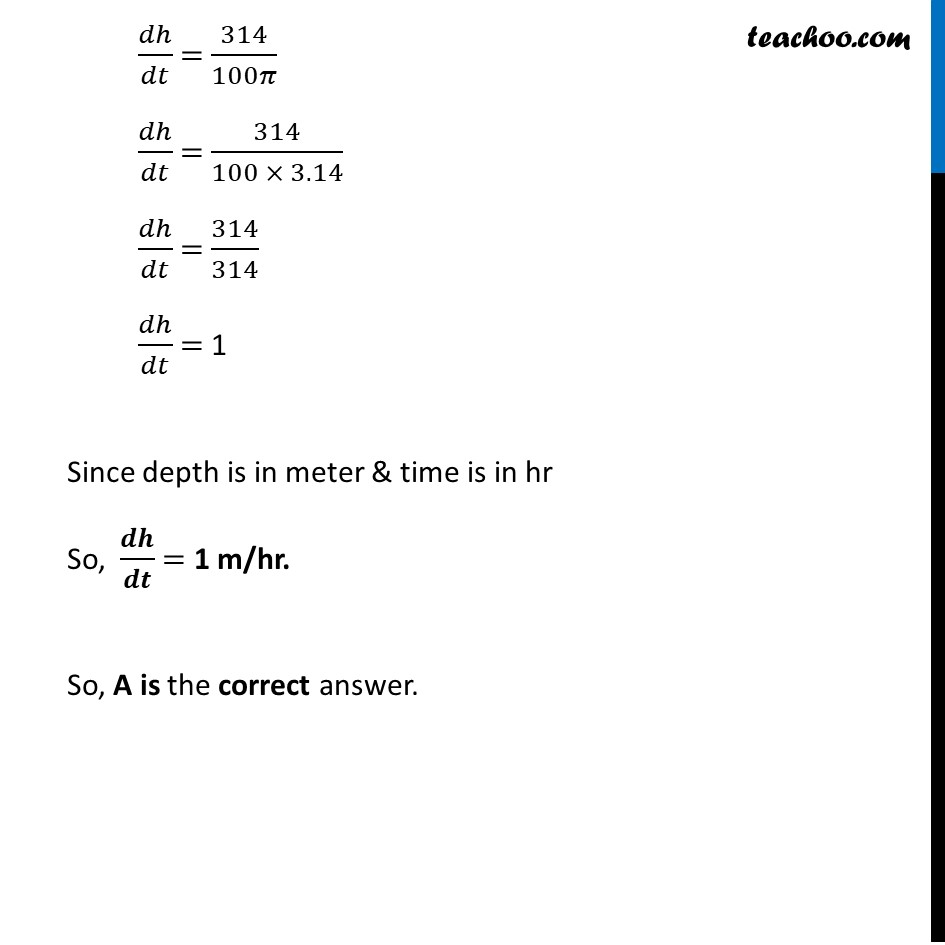Miscellaneous

Chapter 6 Class 12 Application of Derivatives
Serial order wiseLearn in your speed, with individual attention - Teachoo Maths 1-on-1 Class

### Transcript

Misc. 19 A cylindrical tank of radius 10 m is being filled with wheat at the rate of 314 cubic meter per hour. Then the depth of the wheat is increasing at the rate of (A) 1 m /h (B) 0.1 m/h (C) 1.1 m/h (D) 0.5 m/hLet r be the radius of cylindrical tank & V be the volume of cylindrical tank & h be the depth of the cylindrical tank Given that cylindrical tank of radius 10 m being filled with wheat at the rate of 314 cubic meter per hour i.e. Change if volume of tank is 314 m3/hr. when r = 10 i.e. 𝒅𝑽/𝒅𝒕 = 314 m3/hr. when r = 10 We need to find at what rate depth is increasing i.e. we need to find 𝒅𝒉/𝒅𝒕 Now, 𝑑𝑉/𝑑𝑡 = 314 (𝑑 (𝜋𝑟^2 ℎ))/𝑑𝑡 = 314 (𝑑(𝜋(102)ℎ))/𝑑𝑡 = 314 (𝑑(100𝜋ℎ))/𝑑𝑡 = 314 100𝜋 𝒅𝒉/𝒅𝒕 = 314 𝑑ℎ/𝑑𝑡 = 314/100𝜋 𝑑ℎ/𝑑𝑡 = 314/(100 × 3.14) 𝑑ℎ/𝑑𝑡 = 314/314 𝑑ℎ/𝑑𝑡 = 1 Since depth is in meter & time is in hr So, 𝒅𝒉/𝒅𝒕 = 1 m/hr. So, A is the correct answer.